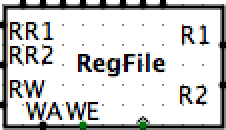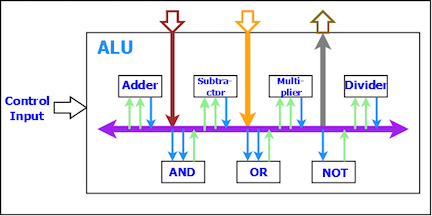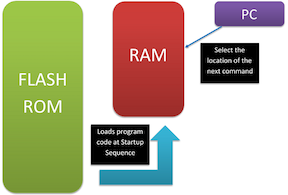# Shixuan Li

0%

Introduction with Definitions and Datapath

## 1. Introduction

This project is based on UC Berkeley, CS61C, Spring 2018, Project 3. The project is revealed by Logisim.

In this project we will be using Logisim to implement a 32-bit two-cycle processor based on RISC-V (Stage1: Single-Cycle; Stage2: Pipelining) The project is mainly consisted by four parts: CPU, PC, RegFile, ALU, RAM. We will first introduce the general definitions and ideas of the project. Then we'll discuss the designing of each part of CPU. Finally talk about the CPU system setup and PipeLining.

### 1.1 Definitions

#### 1.1.1 RISC-VRISC-V (pronounced "risk-five") is an open instruction set architecture (ISA) based on established reduced instruction set computing (RISC) principles. The project began in 2010 at the University of California, Berkeley.

The RISC-V ISA has been designed with small, fast, and low-power real-world implementations in mind, but without over-architecting for a particular microarchitecture style.

Here we pass RISC-V based instructions to our CPU (Real input is converted into Machine code). For more introduction and tutorial for RISC-V, check my related article HERE(Not-Published).

#### 1.1.2 CPUA Central Processing Unit (CPU) is the electronic circuitry within a computer that carries out the instructions of a computer program by performing the basic arithmetic, logical, control and input/output (I/O) operations specified by the instructions. [by Wikipedia].

In this project, we are using a 32-bit CPU, taking machine-code instructions based on RISC-V. You can simply think of a machine, taking in a bunch of zeros'n'ones (or usually we take code in hexadecimal system). Then, we break down the instructions by its "grammar" and reveal their meanings (translation). Then we'll know which operations we need to take to our RAM

#### 1.1.3 RegFileThe functionality of the RegFile is to pass in the new value into the registers and then output the values from the required registers. There are totally 6 input (excluding the registers), and 2 outputs, which we'll explain elaborately later.

#### 1.1.4 ALUA Arithmetic Logic Unit (ALU) is a combinational digital electronic circuit that performs arithmetic and bitwise operations on integer binary numbers.

In short, an ALU here is a functional unit where we can choose and do various arithmetic calculations and return the result.

#### 1.1.5 RAMAn RAM, as a memory, obvious, it is in charge of the storage of values. When we want to load data from memory (lw, lh, lb), we read from RAM; when we want to store data into memory (sw, swge), we store value into RAM.

The RAM is just like a set of boxes that has numbers (address) labeling their identity and contains data. When we want to load data from the RAM, for example, we simply input an address (to specify which box) and output the value inside.

#### 1.1.6 PCA Program Counter (PC) is a processor register that indicates where a computer is in its program sequence.

Note that PC is separate from the 32 operational registers (x0~x31). And since PC indicates the program sequence, we need to adjust the value of PC in order to get our program running the correct instruction. There many special implementations to PC such as when there's an loop or call of another function inside a function (how should we manage the PC? Think about it)

In Part 2 we will be talking about Datapath and Instruction Fetching of the CPU system.